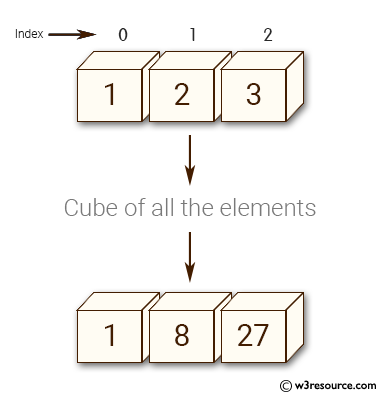﻿ NumPy: Create a function cube which cubes all the elements of an array - w3resource# NumPy: Create a function cube which cubes all the elements of an array

## NumPy: Array Object Exercise-76 with Solution

Write a NumPy program to create a function cube which cubes all the elements of an array.

Pictorial Presentation:Sample Solution:-

Python Code:

``````import numpy as np
def cube(e):
it = np.nditer([e, None])
for a, b in it:
b[...] = a*a*a
return it.operands
print(cube([1,2,3]))
```
```

Sample Output:

```[ 1  8 27]
```

Python Code Editor:

Have another way to solve this solution? Contribute your code (and comments) through Disqus.

What is the difficulty level of this exercise?

Test your Python skills with w3resource's quiz

﻿

## Python: Tips of the Day

Set comprehension:

```>>> m = {x ** 2 for x in range(5)}
>>> m
{0, 1, 4, 9, 16}
```